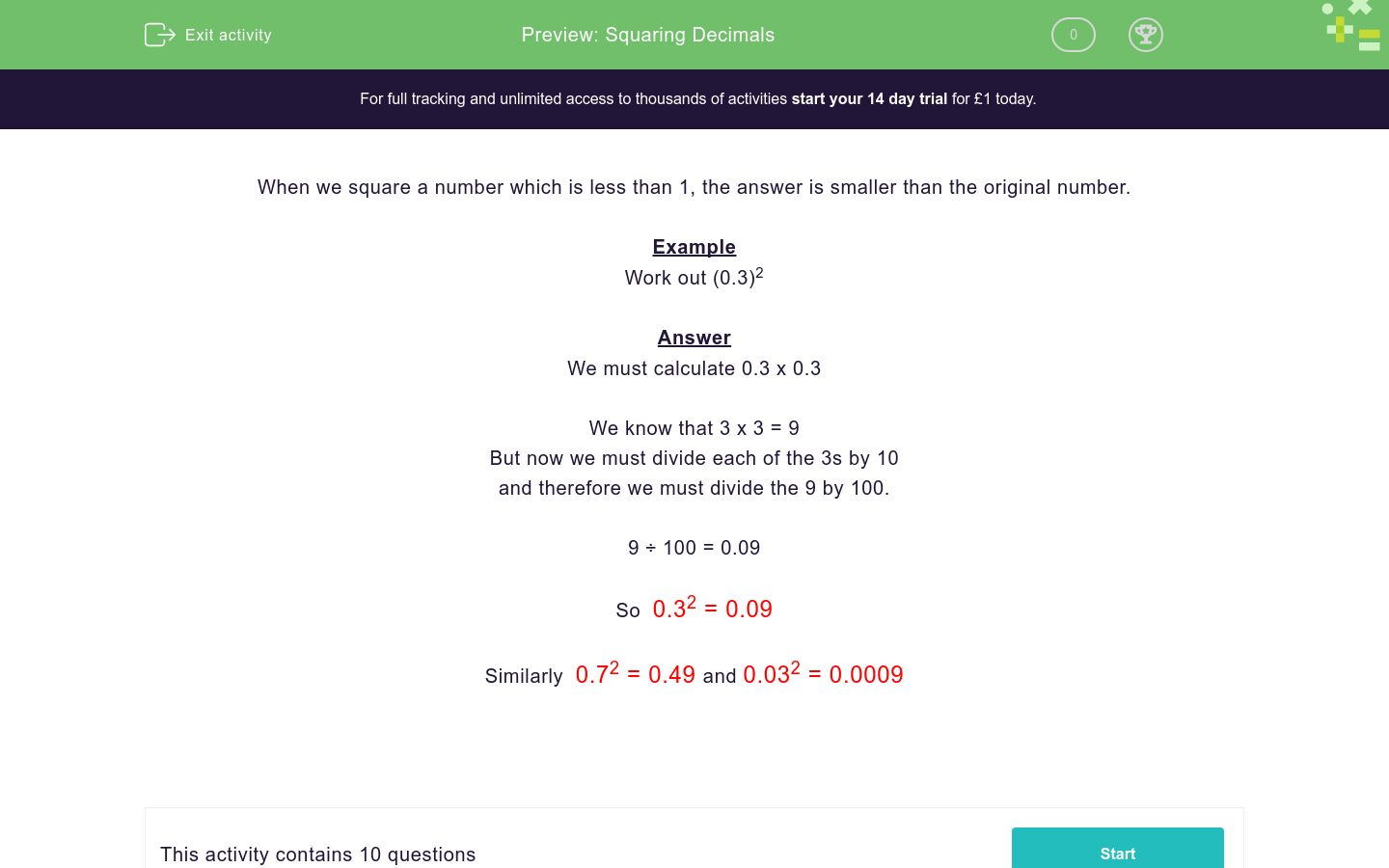# Squaring Decimals

In this worksheet, students square simple decimal numbers.Key stage:  KS 3

Curriculum topic:   Number

Curriculum subtopic:   Understand Integer Powers/Real Roots

Difficulty level:#### Worksheet Overview

When we square a number which is less than 1, the answer is smaller than the original number.

Example

Work out (0.3)2

Answer

We must calculate 0.3 x 0.3

We know that 3 x 3 = 9

But now we must divide each of the 3s by 10

and therefore we must divide the 9 by 100.

9 ÷ 100 = 0.09

So  0.32 = 0.09

Similarly  0.72 = 0.49 and 0.032 = 0.0009

### What is EdPlace?

We're your National Curriculum aligned online education content provider helping each child succeed in English, maths and science from year 1 to GCSE. With an EdPlace account you’ll be able to track and measure progress, helping each child achieve their best. We build confidence and attainment by personalising each child’s learning at a level that suits them.

Get started#### Similar activities you may like

Try an activity or get started for free